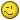# Sum of row and column entries (database)

C

#### christopher.j.denver

Hi newsgroup,

I was wondering whether anyone knows a formula that does the
following:

I have a number of values in a column and row of a large database that
i want to sum automatically, as in the following example:

x1 x2 x3 x4 x5
y1 (x1+y1) (x2+y1) (x3+y1) (x4+y1) (x5+y1)
y2 (x1+y2) (x2+y2) (x3+y2) (x4+y2) (x5+y2)
y3 (x1+y3) (x2+y3) (x3+y3) (x4+y3) (x5+y3)
y4 (x1+y4) (x2+y4) (x3+y4) (x4+y4) (x5+y4)
y5 (x1+y5) (x2+y5) (x3+y5) (x4+y5) (x5+y5)

I think I might solve this with a Pivot table, but I have very little
knowledge of pivots...

Thanks a lot and best regards,

Chris

D

#### David Biddulph

If you've got your X values in row 1 in B1 to F1, and your Y values in
column A in A2 to A6, then in cell B2 insert the formula =B1+A2. Then copy
that cell to the other cells down and across your range.

C

#### christopher.j.denver

Hi David,
If you've got your X values in row 1 in B1 to F1, and your Y values in
column A in A2 to A6, then in cell B2 insert the formula =B1+A2. Then copy
that cell to the other cells down and across your range.

Thanks, but this doesn't really do it, as the copying across the sheet
results in Excel changing the cell references accordingly. I could do
it for each column by using the F4 command (fixing cells like \$A\$1),
but even this is quite lengthy, as I have more than 200 columns and
rows to fill...

Regards,

Chris

D

#### David Biddulph

Sorry, yes, you are absolutely right. I was half asleep.
What I should have said was not =B1+A2 but =B\$1+\$A2
That will fix the row 1 and column A references accordingly, and allow the
rest to update.

C

#### christopher.j.denver

Hi David,
Sorry, yes, you are absolutely right. I was half asleep.
What I should have said was not =B1+A2 but =B\$1+\$A2
That will fix the row 1 and column A references accordingly, and allow the
rest to update.

Amazing, it's that easyThanks a lot, this has saved me hours of manually adjusting it - it
never crossed my mind that it's so straight to adjust the "fixing \$"
thoughThanks again,

Chris